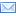Difference Between Similar Terms and Objects

Difference Between Null and Alternative HypothesisNull vs Alternative Hypothesis

A hypothesis is described as a proposed explanation for an observable phenomenon. It is intended to explain facts and observations about the natural world, providing insight that has not been verified but can be proven true. It is a prediction of a possible outcome and describes what will happen. It involves research and may be part of a theory or may become a theory. There are several types of hypothesis:

* Inductive and Deductive Hypothesis. An inductive hypothesis is based on general observations while a deductive hypothesis is based on theory and either supports, expands, or contradicts the theory.
* Directional and Non-directional Hypothesis. A directional hypothesis states the expected direction of the relation or difference while a non-directional hypothesis states that a relation or difference exists between variables.
* Simple and Composite Hypothesis. A simple hypothesis concerns the population as a functional form and parameter. If it is not simple, then it is a composite hypothesis.
* Parametric and Non-Parametric Hypothesis. A parametric hypothesis specifies the parameters of the probability density function while a non-parametric hypothesis specifies only the form of the density function in the population.
* Null and Alternative Hypothesis. A null hypothesis is a statistical hypothesis and is the default or original hypothesis while an alternative hypothesis is any hypothesis other than the null. If the null hypothesis is not accepted, then the alternative hypothesis is used. H0 is a null hypothesis while H1 is an alternative hypothesis.

Research studies and testing usually formulate two hypotheses. One will describe the prediction while the other will describe all other possible outcomes. For example, you predict that A is related to B (null hypothesis). The only other possible outcome is that they are not related (alternative hypothesis).

Although the alternative hypothesis can be negative, it is not necessarily a negation of the null hypothesis but rather a gauge of whether the null hypothesis is true or not. It is an essential component of statistical hypothesis testing and is generally accepted and used in modern statistical studies.

A null hypothesis, on the other hand, is used in scientific and medical research to test the differences in treatment and control groups. It predicts that there is no difference between the control and experimental groups, but it can have other possibilities.

Another example of the difference between a null hypothesis and an alternative hypothesis is in the legal system. The original hypothesis is that the defendant is innocent until he is proven guilty. His innocence is the null hypothesis while his guilt is the alternative hypothesis.

Summary:

1. A null hypothesis is a statistical hypothesis which is the original or default hypothesis while any other hypothesis other than the null is called an alternative hypothesis.
2. A null hypothesis is denoted by H0 while an alternative hypothesis is denoted by H1.
3. An alternative hypothesis is used if the null hypothesis is not accepted or rejected.
4. A null hypothesis is the prediction while an alternative hypothesis is all other outcomes aside from the null.
5. Both the null and alternative hypotheses are necessary in statistical hypothesis testing in scientific, medical, and other research studies.

Search DifferenceBetween.net :

Custom Search

Help us improve. Rate this post!(7 votes, average: 4.43 out of 5)Loading...Email This Post : If you like this article or our site. Please spread the word. Share it with your friends/family.

1. what are the alternative hypotheses

2. please give a problem as a example…

3. What is the null hypothesis plz Explain it to me with Example ?? Or Email to me the Explanation Sherwaheed56@Gmail.com I waiting ur answer plzz??

Please note: comment moderation is enabled and may delay your comment. There is no need to resubmit your comment.

Articles on DifferenceBetween.net are general information, and are not intended to substitute for professional advice. The information is "AS IS", "WITH ALL FAULTS". User assumes all risk of use, damage, or injury. You agree that we have no liability for any damages.